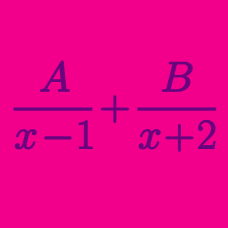Algebra

# Partial Fractions - Irreducible Quadratics

If the following is an identity in $x$: $\frac{9x^2+9}{x^3+1}=\frac{a}{x+1}+\frac{bx+c}{x^2-x+1},$ what is the value of $a+b+c$?

If the following is an identity in $x$: $\frac{10x^2+1}{x(x^2+1)}=\frac{a}{x}+\frac{bx+c}{x^2+1},$ what is the value of $a+b+c$?

If the following is an identity in $x$: $\frac{9x-4}{x^3-2x+1}=\frac{a}{x-1}+\frac{bx+c}{x^2+x-1},$ what is the value of $a \times b \times c$?

If the following is an identity in $x$: $\frac{5x^2+Ax+B}{(7x+1)(x^2-7x+1)}=C\left(\frac{1}{7x+1}+\frac{1}{x^2-7x+1}\right),$ what is the value of $A+B+C?$

Which of the following is the partial fraction decomposition of

$\frac{ 1} { x^3 + x } ?$

×